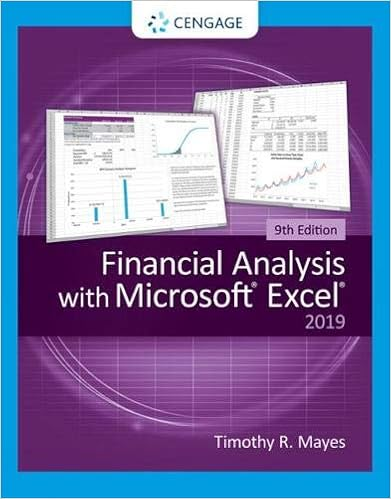# On hurricane chuck destroyed bedford craft marts

• Notes
• shaker2412
• 184
• 93% (14) 13 out of 14 people found this document helpful

This preview shows page 40 - 50 out of 184 pages.

##### We have textbook solutions for you!
The document you are viewing contains questions related to this textbook.The document you are viewing contains questions related to this textbook.
Chapter 2 / Exercise 2
Financial Analysis with Microsoft Excel
Mayes/ShankExpert Verified
95.On August 31, 2013, Hurricane Chuck destroyed Bedford Craft Mart's entire inventory. The following information is available from its accounting records:Required:Assuming that Bedford estimates the cost of destroyed inventory at \$510,000, compute gross profit margin % that Bedford uses in estimating inventory.
##### We have textbook solutions for you!
The document you are viewing contains questions related to this textbook.The document you are viewing contains questions related to this textbook.
Chapter 2 / Exercise 2
Financial Analysis with Microsoft Excel
Mayes/ShankExpert Verified
96.Andover Stores uses the average cost retail method to estimate its ending inventory. Information as of June 30, 2013, is as follows:Required:Use the retail method to estimate the June 30, 2013, inventory.
97.DK Super Stores Inc. uses the average cost retail method to estimate its ending inventory. Information at June 30, 2013, is as follows:Required:Compute the cost-to-retail percentage used by DK.
98.Trask Inc. uses the average cost retail method to estimate its ending inventory. Partial information at June 30, 2013, is as follows:Required:Assuming Trask's cost-to-retail = 60%, compute Trask's beginning inventory at retail.
99.Manila Bread Company uses the average cost retail method to estimate its ending inventories. The following data has been summarized for the year 2013:Required:Estimate the ending inventory as of December 31, 2013.
100.Penfold's Paints uses the average cost retail method to estimate its ending inventories. The following data has been summarized for the year 2013:Required:Compute the cost-to-retail percentage used by Penfold's Paints.
101.Murdock Industries uses a periodic inventory system and the LIFO retail method to estimate its ending inventories. The following data has been summarized for December 31, 2013:Required:Estimate the LIFO cost of ending inventory. Assume stable retail prices during the period.
102.Littleton Company uses a periodic inventory system and the LIFO retail method to estimate its ending inventories. The following partial data has been summarized for December 31, 2013:Required:Determine the cost-to-retail percentage used by Littleton. Assume stable retail prices during the period.
103.Billingsly Products uses the conventional retail method to estimate its ending inventories. The following data has been summarized for the year 2013:Required:Estimate the ending inventory as of December 31, 2013.
104.New York Sales Inc. uses the conventional retail method to estimate its ending inventories. The following data has been summarized for December 31, 2013:Required:Compute the cost-to-retail percentage used by New York Sales Inc.
•••